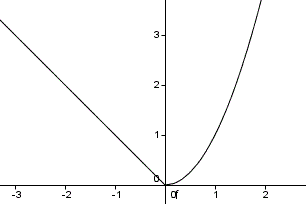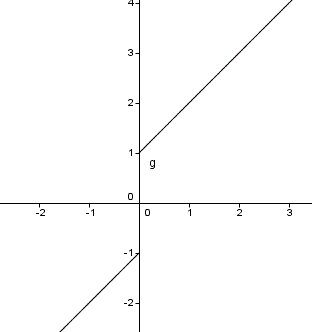# Non Differentiable Functions

Questions on the differentiability of functions with emphasis on piecewise functions are presented along with their answers.

 Graphical Meaning of non differentiability. Which Functions are non Differentiable? Let f be a function whose graph is G. From the definition, the value of the derivative of a function f at a certain value of x is equal to the slope of the tangent to the graph G. We can say that f is not differentiable for any value of x where a tangent cannot 'exist' or the tangent exists but is vertical (vertical line has undefined slope, hence undefined derivative). Below are graphs of functions that are not differentiable at x = 0 for various reasons. Function f below is not differentiable at x = 0 because there is no tangent to the graph at x = 0.(try to draw a tangent at x=0!)Function g below is not differentiable at x = 0 because there is no tangent to the graph at x = 0.(try to draw a tangent at x=0!)Function h below is not differentiable at x = 0 because there is a jump in the value of the function and also the function is not defined therefore not continuous at x = 0.Function j below is not differentiable at x = 0 because it increases indefinitely (no limit) on each sides of x = 0 and also from its formula is undefined at x = 0 and therefore non continuous at x=0 .Function k below is not differentiable because the tangent at x = 0 is vertical and therefore its slope which the value of the derivative at x =0 is undefined.Theorem: If a function f is differentiable at x = a, then it is continuous at x = a Contrapositive of the above theorem: If function f is not continuous at x = a, then it is not differentiable at x = a. Common mistakes to avoid: If f is continuous at x = a, then f is differentiable at x = a. NOTE: Although functions f, g and k (whose graphs are shown above) are continuous everywhere, they are not differentiable at x = 0. Analytical Proofs of non differentiability Example 1: Show analytically that function f defined below is non differentiable at x = 0. f(x) = \begin{cases} x^2 & x \textgreater 0 \\ - x & x \textless 0 \\ 0 & x = 0 \end{cases} Solution to Example 1 One way to answer the above question, is to calculate the derivative at x = 0. We start by finding the limit of the difference quotient. Since function f is defined using different formulas, we need to find the derivative at x = 0 using the left and the right limits. f'(x) = \lim_{h\to\ 0} \dfrac{f(x+h) - f(x)}{h} On the left of x = 0 (x < 0), the derivative is calculated as follows f'(0) = \lim_{h\to\ 0^-} \dfrac{f(0+h) - f(0)}{h} = \lim_{h\to\ 0} \dfrac{ -h - 0}{h} = -1 On the right of x = 0 (x > 0), the derivative is calculated as follows f'(0) = \lim_{h\to\ 0^+} \dfrac{f(0+h) - f(0)}{h} = \lim_{h\to\ 0} \dfrac{h^2 - 0}{h} = \lim_{h\to\ 0} h = 0 The limits to the left and to the right of x = 0 are not equal therefore f '(0) is undefined and function f in not differentiable at x = 0. More on Continuous Functions in Calculus Continuity Theorems and Their use in Calculus Questions on Continuity with Solutions. Home Page